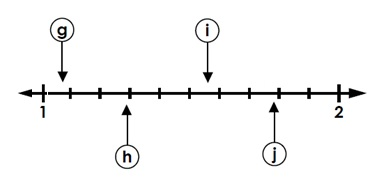# Reading Decimal Position on a Number Line Hundredths Online Quiz

#### Complete Python Prime Pack

9 Courses     2 eBooks

#### Artificial Intelligence & Machine Learning Prime Pack

6 Courses     1 eBooks

#### Java Prime Pack

9 Courses     2 eBooks

Following quiz provides Multiple Choice Questions (MCQs) related to Reading Decimal Position on a Number Line Hundredths. You will have to read all the given answers and click over the correct answer. If you are not sure about the answer then you can check the answer using Show Answer button. You can use Next Quiz button to check new set of questions in the quiz.Q 1 - The letter b on the number line represents the decimalA - 3.5

B - 0.35

C - 3.035

D - 35.0

### Explanation

Step 1:

The number line is divided into hundredths between 0 and 1

Step 2:

The letter b on the number line points to the decimal thirty-five hundredths or 0.35

Q 2 - The letter i on the number line represents the decimalA - 1.57

B - 0.57

C - 15.7

D - 0.057

### Explanation

Step 1:

The number line is divided into hundredths between 1 and 2

Step 2:

The letter i on the number line points to the decimal one and fifty-seven hundredths or 1.57

Q 3 - The letter c on the number line represents the decimalA - 0.042

B - 0.44

C - 4.2

D - 0.42

### Explanation

Step 1:

The number line is divided into hundredths between 0 and 1

Step 2:

The letter c on the number line points to the decimal forty-two hundredths or 0.42

Q 4 - The letter g on the number line represents the decimalA - 1.8

B - 1.18

C - 1.08

D - 1.10

### Explanation

Step 1:

The number line is divided into hundredths between 1 and 2

Step 2:

The letter g on the number line points to the decimal one and eight hundredths or 1.08

Q 5 - The letter e on the number line represents the decimalA - 0.78

B - 0.078

C - 7.8

D - 78.00

### Explanation

Step 1:

The number line is divided into hundredths between 0 and 1

Step 2:

The letter e on the number line points to the decimal seventy-eight hundredths or 0.78

Q 6 - The letter j on the number line represents the decimalA - 0.79

B - 1.079

C - 1.79

D - 17.9

### Explanation

Step 1:

The number line is divided into hundredths between 1 and 2

Step 2:

The letter j on the number line points to the decimal one and seventy-nine hundredths or 1.79

Q 7 - The letter a on the number line represents the decimalA - 0.016

B - 0.16

C - 1.6

D - 16.0

### Explanation

Step 1:

The number line is divided into hundredths between 0 and 1

Step 2:

The letter a on the number line points to the decimal sixteen hundredths or 0.16

Q 8 - The letter h on the number line represents the decimalA - 2.9

B - 29.0

C - 0.29

D - 1.29

### Explanation

Step 1:

The number line is divided into hundredths between 1 and 2

Step 2:

The letter h on the number line points to the decimal one and twenty-nine hundredths or 1.29

Q 9 - The letter d on the number line represents the decimalA - 0.54

B - 0.054

C - 5.4

D - 54.0

### Explanation

Step 1:

The number line is divided into hundredths between 0 and 1

Step 2:

The letter d on the number line points to the decimal fifty-four hundredths or 0.54

Q 10 - The letter f on the number line represents the decimalA - 9.1

B - 0.091

C - 0.91

D - 91.0

### Explanation

Step 1:

The number line is divided into hundredths between 0 and 1

Step 2:

The letter f on the number line points to the decimal ninety-one hundredths or 0.91